Courses

# Test: Aromaticity And Acid Base Properties

## 15 Questions MCQ Test Chemistry Class 11 | Test: Aromaticity And Acid Base Properties

Description
This mock test of Test: Aromaticity And Acid Base Properties for Class 11 helps you for every Class 11 entrance exam. This contains 15 Multiple Choice Questions for Class 11 Test: Aromaticity And Acid Base Properties (mcq) to study with solutions a complete question bank. The solved questions answers in this Test: Aromaticity And Acid Base Properties quiz give you a good mix of easy questions and tough questions. Class 11 students definitely take this Test: Aromaticity And Acid Base Properties exercise for a better result in the exam. You can find other Test: Aromaticity And Acid Base Properties extra questions, long questions & short questions for Class 11 on EduRev as well by searching above.
QUESTION: 1

### Direction (Q. Nos. 1 - 10) This section contains 10 multiple choice questions. Each question has four choices (a), (b), (c), and (d), out of which ONLY ONE option is correct. Q. Arrange the following compounds in increasing order of their basic strength.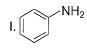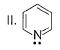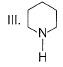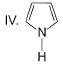Solution: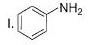Aniline (pKvalue = 4.87)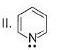Pyridine (pKvalue = 5.2)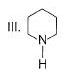Piperidine (pKvalue = 11.22)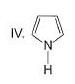Pyrrole ( pKvalue =16.5)

Higher the pKa value (lower acidity), higher will be the basicity.

So the order of basic strength will be

I < II < III < IV

QUESTION: 2

Solution:
QUESTION: 3

### Which of the following compounds is more easily oxidised to a carbonyl when treated with MnO2?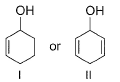Solution:

MnO2 is used for the selective oxidation of allylic and benzylic carbon and in these compounds these carbons are not very easily oxidisable.

QUESTION: 4

Which among these is the simplest example for polycyclic arenes?

Solution:

Naphthalene has fused ring of aromaticity and has the simplest structure when compared with other polycyclic aromatic hydrocarbons.

QUESTION: 5

The compound shown below evolve hydrogen gas when refluxed with potassium metal, why?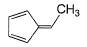Solution:
QUESTION: 6

Which compound below has maximum tendency to form a salt when treated with HBr?

Solution:
QUESTION: 7

What is the correct order of increasing acidic strength of the following?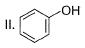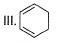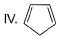Solution:
QUESTION: 8

How many Kekule structures exists for naphthalene?

Solution:

We'll use the three double bond symbol simply because it is also routinely used in the text. Keep in mind that if the hexagon contains neither the three double bonds nor the circle, the compound is not aromatic. It is simply cyclohexane and there are two hydrogens on each carbon atom.

QUESTION: 9

How many monobromo derivatives exists for anthracene?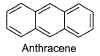Solution:

The correct answer is Option B.

There are 3 monobromo derivatives exists for anthracene:
1-Chloroanthracene
2-Chloroanthracene
and 9-Chloroanthracene

QUESTION: 10

7-bromo-1,3,5-cycloheptatriene exists as ionic species in aqueous solution while 5-bromo-1,3-cyclopentadiene does not ionise even in presence of AgNO3(aq) because

Solution:

The correct answer is Option C.
The C-Br bond in the case of 7-bromo-1, 3, 5-cycloheptatriene is broken easily because the intermediate carbocation formed is very stable (aromatic as it contains (4n + 2)π e- ie, follows Huckle rule) while it does not break easily in the case of S-bromo-1, 3-cyclopentadiene because carbocation formed here is highly unstable as it is anti aromatic ie, does not follow Huckel rule. (It contains 4π electrons).

*Multiple options can be correct
QUESTION: 11

Direction (Q. Nos. 11-15) This section contains 5 multiple choice questions. Each question has four choices (a), (b), (c) and (d), out of which ONE or MORE THAN ONE are correct.

Q. Consider the following compounds.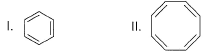The correct statement regarding properties of above mentioned compounds is/are

Solution:
*Multiple options can be correct
QUESTION: 12

Which of the following systems are aromatic?

Solution:

The correct answers are Options C and D.
Aromatic compounds are those which follow Huckel's rule i.e, they have (4n+2) π electrons, n must be an integer.
In option C, there are 5 π bonds which means 10 π electrons; so 4n+2 = 10 i.e, n= 2 which is an integer.
In option D, Nitrogen has a lone pair which contains 2 electrons therefore this compound also have 10 π electrons; so n= 2

*Multiple options can be correct
QUESTION: 13

What is true about the 1,3,5,7-cyclooctatetraene?

Solution:
*Multiple options can be correct
QUESTION: 14

What is true regarding the following compound?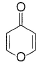Solution:

The given compound will turn itself to
To gain aromaticity, it will transfer the electrons as follow:-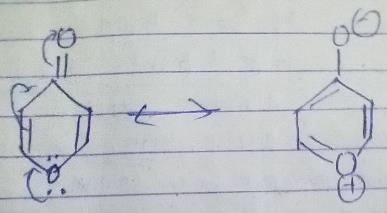It is clear that the compound is heterocyclic(the ring constitutes other than C and H). Due to -ve charge on outer O atom, it has high affinity for BF3. However, NaBH4  has no reaction with this. As the cound will turn itself to latter, there is no aldehyde or ketone group present in the compound.

QUESTION: 15

Organic compounds can be classified even based upon the function groups. Identify the one which is not a functional group

Solution:

Isocyanide is a compound and it is not a functional group.